# Excel tip: Suppress zero values on summary worksheets

## Microsoft Excel consultant Bill Jelen, aka "MrExcel," shows how to prevent zeros from displaying in a summary worksheet.

Frequently, a complex Microsoft Excel workbook will use several worksheets that need to feed a summary workbook....

A problem happens when the original worksheet contains sparse data, such as the data shown in Figure 1.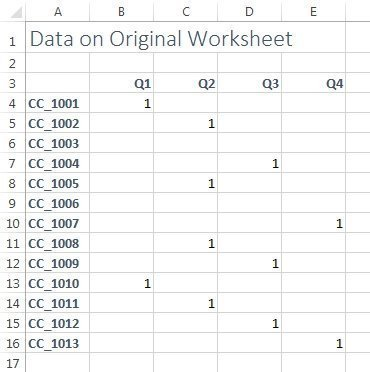Figure 1

When you use a simple formula to pull that range from the original worksheet to a summary worksheet, all the empty cells turn into zeros in the summary worksheet.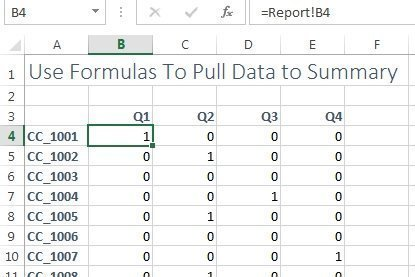Figure 2

However, there are several ways of making sure that the zeros do not appear in the summary worksheet.

Instead of =Report!B4, you could use an IF formula to check if the original cell is blank, such as the formula =IF(ISBLANK(Report!B4),"",Report!B4). This formula checks to see if the source cell is blank. If the original cell is empty, the formula will show a blank thanks to the "" in the formula. The non-blank cells copy the original cell.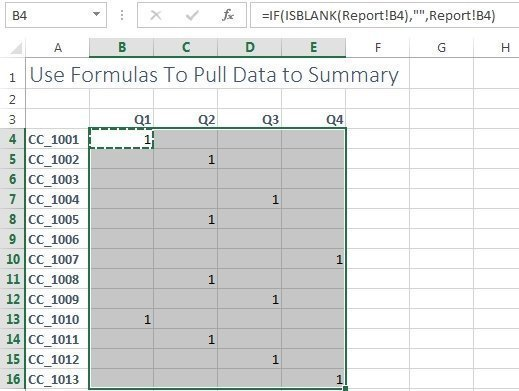Figure 3

Another option is to allow the zeros to appear, but to suppress the display of zeros. To set this up, follow these steps:

2. From the left navigation, choose Options.
3. In the Excel Options dialog, click Advanced in the left navigation.
4. Scroll 60% of the way through the advanced Excel options. Look for the section called "Display options for this worksheet." In that section, uncheck the checkbox for "Show a zero in cells that have zero values."
5. Click OK to close Excel Options.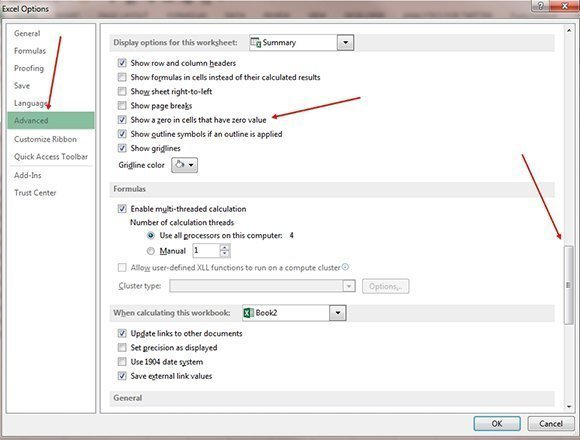Figure 4

Now, although the zeros are still there, Excel will not display or print them.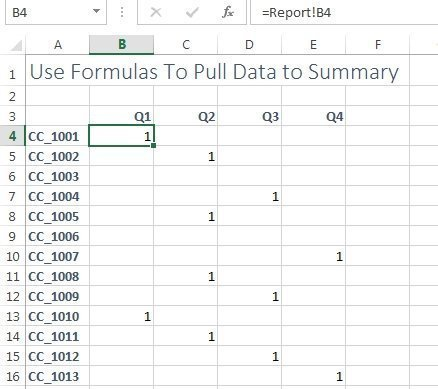Figure 5

The setting in Figure 3 applies to all cells on the worksheet. If other sections on the summary need to display zeros, you can suppress them in a particular range using a Custom Number Format code:

1. Select the range where you want to suppress zeros.
2. Press Ctrl+1 to display the Format Cells dialog.
3. Click the Number tab.
4. In the Category box, choose Custom.
5. In the Type box, use 0;-0;;
6. Click OK.

The custom number format code allows for different zones, separated by semicolons. Because this code has three semicolons, there are four zones -- one for positive, one for negative, one for zero and one for text.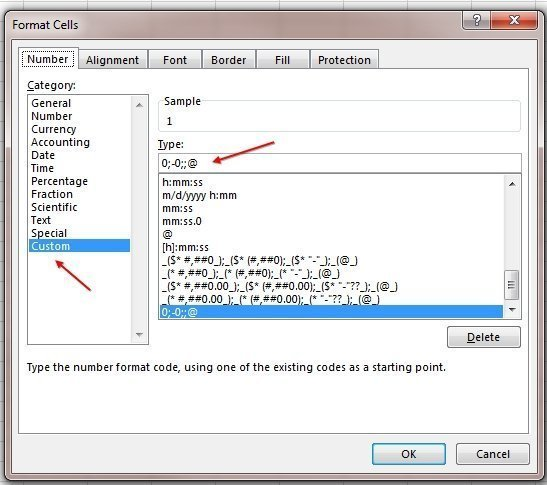Figure 6

Nothing is in the third and fourth zone of the custom number format, so any zero or text values are not displayed.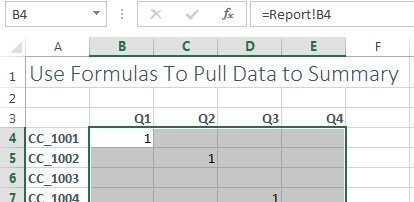Figure 7

Several Excellers have been asking Microsoft to someday offer an EMPTY() function to return true empty cells as the result of an IF function. Until that happens in Excel 2025, the methods in this article allow you to work around the problem of blanks changing to zeros.

Bill Jelen, aka "MrExcel," has been a Microsoft Excel consultant for more than 25 years. Read more of his expert Excel tips and tricks at his website, MrExcel.com.

Close